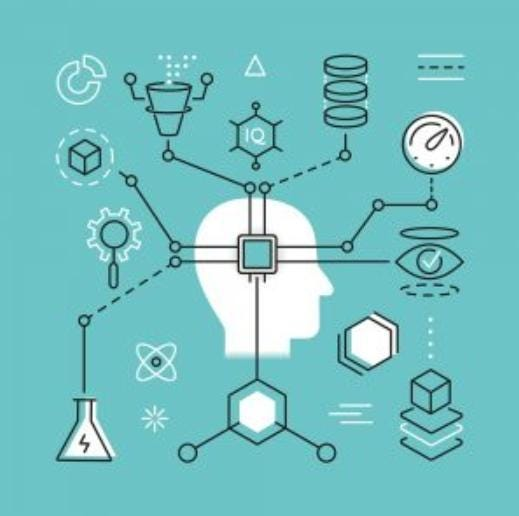# Introduction to Machine learning# BLACK-BOX VS INFERENCE

Models which are only used predictions are called black-box but when we are interested in evidences and reasons behind it , it is called as inference . If we want the inference then we need to know the relationships between inputs and outputs . Some of black-box algorithms are discriminant analysis , KNN , neural networks etc . Inference algorithms are OLS, best subset regression, penalised regression, logistic regression .

# RESTRICTIVE MODELS VS FLEXIBLE MODELS

Some of the models only allows limited numbers of shapes for functions . 1. linear regression — only linear graphs . 2. logistic regression — only linear graphs . These are called restrictive models . There are some models which allows many different shapes of functions 1. support vector machine — linear, polynomial etc..2. neural networks — various types .

# ESTIMATION ERROR

Sometimes we need to know how are model is working . here, estimation error comes to work . estimation is done by : X-Y . X is the actual value given in the dataset and Y is the estimated value(prediction value) . it is better if the estimation error is small . for regression, we use MSE(Mean Squared Error) , R²(R Squared error) and Adjusted R² . for classification ,we us confusion matrix .

MSE

Here, this is the formula of mean squared error . n is the sample size , yi is the actual value and y~ is the estimated value .

R² error

this is the formula of r² . if r² is equal to 0.80, 80% of the variation is the response variable is determined by the predictors .

Confusion matrix

The prediction accuracy of a classification model is given by the percentage of the correctly classified cases . To compute the cases ,we use the confusion matrix . Here, the True Positives are the one which are positives and the prediction is right(true) . False Positives are the one which are positives but the prediction are wrong(false) . False Negatives are the one which are negatives but the prediction is wrong(false) . True Negatives are the one which are negatives and the prediction is right(true) .

# BIAS — VARIANCE TRADE OFF

Bias is how far the predicted values are from the actual value . Variance is how scattered the predicted values are from actual values .

Total error = Bias + Variance

Low bias and low variance are better . High variance = under fitting . High bias = over fitting .

# CROSS VALIDATION

Cross validation is the technique meant how to access how well a machine learning model performs on the first seen data . We get the accuracy of the model on first seen data . 1. Validation Set approach randomly splits the data into train and test data . 2. Leave-one-out cross validation (LOOCV) it samples and fits the model on other observations . 3. k-fold cross validation it randomly splits the overall data . but first on model is retained as test set then the k-1 subsamples .